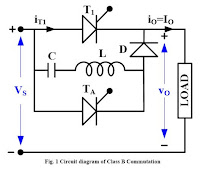Resonant Pulse commutation is also called current commutation, forced commutation, and Class-B commutation. It is used in dc choppers and inverters.
• Source voltage VS charges capacitor C to voltage VS
• At t = t1, TA is ON, a resonant current iC begins to flow through C, TA, L and back to C.
• ic = -VS*sqrt(C/L)*Sin(OMEGAO*t)
• ic = -IP*Sin(OMEGAO*t)
• vc = 1/C*integration*(ic)*dt
• vc = VS*Cos(OMEGAO*t)Add caption
• At t = t2: ic = 0, vc = -VS, iT1 = IO
• Now ic builds up through C, L, D, and T1.
• iT1=Io-iC
• At iC=Io, iT1=Io-Io=0
• T1 is turned OFF at t=t3
• For reliable commutation, Peak resonant current I> Io
• As thyristor is commutated by the gradual buildup of resonant current in the reversed direction,  method is called current commutation or resonant pulse commutation.
• At t = t3, vc = - Vab, Io flows from Vs to load through C, L and D.
• At t = t4, vc = 0
• At t = t5, vc = Vs, io = ic = 0
• When T1 is turned OFF, Vs*sqrt(C/L)*sin(omegao(t3-t2))=Io
• Omegao(t3-t2))=Invsin(Io/Ip)
• Circuit turn OFF time for main thyristor, tc=t4-t3=C*Vab/Io
• Vab=Vs*cos(Omegao(t3-t2))

#### Reference

Dr. P. S. Bimbhra, "Power Electronics", Khanna Publishers, Fifth Edition, 2013.